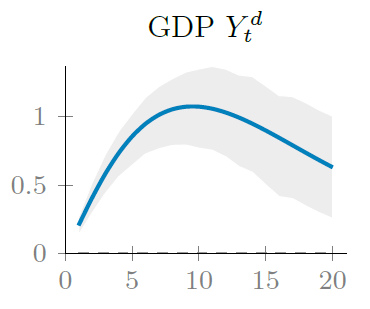The lectures provide a self contained introduction to the building, simulation and estimation macroeconometric models that constitute the main workhouse of today’s macroeconomics. These models are now commonly used in central banks for policy projections and business cycle analysis. Their interest is to mix micro foundations, dynamic relations and rational expectations. Furthermore, they can be estimated using recent developments in Bayesian econometrics. The objective of this course is to provide the both basic and advanced tools to allow participants to get some intuitions on these models and to be able to read and understand policy and research papers using this approach. Lectures are followed by practical sessions with hands-on computational exercises.

Installing MATLAB: Dauphine provides a free licence for students. Please use your own laptop if you can (with MATLAB installed prior to the lectures). Datafeed toolbox and optimization are required, make sure you have them installed.

# Real time macro-data on MATLAB

This is a must-read as all of the lectures will rely on DBnomics. The latter is a database aggregator which can be queried directly from MATLAB. Please read carefully this following note on DBnomics and download as well the MATLAB function which allows to get real time macro data:

# Papers presentations 2018/2019

 Name Paper Matteo & Alex GK model of unconventional monetary policy tbd tbd

# Handouts list

Lecture 1: An Introduction to Dynare Programming
Objectives of the Lecture:

• Introducing to the simulation of a very simple business cycle model.
• Solving any theoretical model, find its steady state, log-linearise it and plot its IRFs.
• Computing second moment statistics by hand using artificial series.

Materials:

Lecture 2: Estimation of the Neo-Classical Growth Model
Objectives of the Lecture:
• Estimating the neo-classical growth model through likelihood methods;
• Incoporating trends and estimating them;
• Estimating multiple shocks;
• Ranking estimated models using a log-likelihood criterion;

Materials:

Lecture 3: Perturbation Methods and the Full-fledged RBC Model
Objectives of the Lecture:
• Understanding the real business cycle theory and its theoretical foundations regarding consumption smoothing, asset price fluctuations etc.
• Developing different models of asset pricing.
• Performing higher order approximations to the model’s policy function (second or third order IRF).
• Estimating the RBC model with four time series.

Materials:

Lecture 4: New Keynesian Macroeconomics and Bayesian Econometrics
Objectives of the Lecture:
• Mastering the theoretical foundations of the New Keynesian framework;
• Understanding the relationship between VAR and DSGE models;
• Estimating a DSGE model using Bayesian techniques;

Materials:

Lecture 5: Optimal Fiscal and Monetary Policies
Objectives of the Lecture:
• Incorporating monetary and fiscal policy in a business cycle framework;
• Evaluating the effectiveness of policy using a loss function;
• Performing a welfare ranking of alternative macroeconomic policies;

Materials: